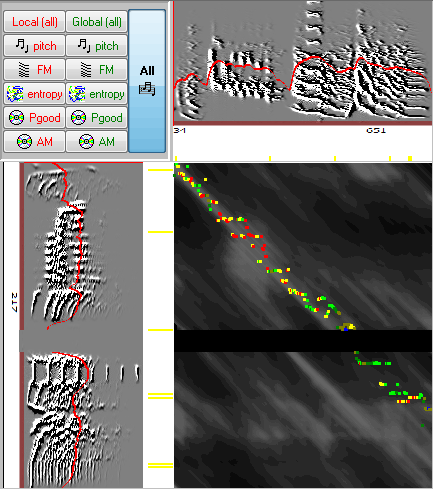# Symmetric measurements

 < PREVIOUS: Asymmetric measurements______________________________________ > NEXT: Time course and mean values

In symmetric comparisons, the similarity is estimated from the beginning of the two sounds. Comparison is done on the 450 of the matrix (diagonal). However, we allow  "tolerances levels" (as if the the diagonal has some 'thickness) withing which we select the highest similarity score. The user can control with the ‘min dur’ slider.

The symmetric measurements are very simple, those are the mean values of similarity scores across the diagonal line. As before, we use both global and local scale, where the global scale is called 'similarity' and the local scale is called 'accuracy':

%similarity: the mean global similarity score (usually across 50ms time windows) across the diagonal with tolerance set by 'min dur'

%accuracy: the mean local similarity score (at the millisecond level) across the diagonal with tolerance set by 'min dur'

%Sequential match: note that the shortest interval selected by the user (in sound 1 or sound 2) determine the intervals of comparisons. The ratio between the two intervals is shown in 'sequential match'. Namely, it is min(duration1,duration2)/max(duration1,duration2). It is not a similarity estimate here, just an indicator for how much of the outlined intervals were actually compared.

A typical symmetric comparison is done at the syllable level, e.g., take a look at this asymmetric comparison of these sounds:There are two sections of similarity that form parallel lines with a gap. In symmetric comparison (and no tolerance) the comparison is strictly on the diagonal:and adding 15ms tollerance looks like this:and we can see that the similarity is higher in the first part.

Note that in symmetric comparison reversing the order of Example 1 and Example 2 should not affect the similarity values (including % similarity).

To get the symmetric comparison to behave properly in this case, we need to manually segment the syllable in Example 2:
So, when should one use symmetric comparisons? These measurements are the best choice if we know in advance the sound units that should be compared and if we do not categorize one sound as a model for the other. For example, comparing two calls of the same type across different members of a colony calls for symmetric scoring. Also, once we have identified a cluster of syllables we might want to measure the similarity across sounds of this cluster, here too, a symmetric approach is most appropriate. SAP2011 provides a method for automatically identifying clusters across files and scoring their similarity (allowing batching of many thousands of comparisons, see batch operations for details).